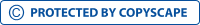Mod2 SLP: Financial decision makingView larger

# Mod2 SLP: Financial decision making

543141

• High School
• 501

Short excerpt:

Question#1 S= 3800000 S= Future Value P= ?P= Present Value r= 5% r= interest rate t= 1 yr t= time S=P(1+rt) 3800= P(1+(0.05)(1)) P= \$3619 Question#2 Account A Account B S= 4100 S= 5500 P= ?t= 2 yrs t= 1 yr P= ?r= 5% r= 5% S=P(1+rt) S=P(1+rt) 4100= P(1+(0.05)(1)) 5500= P(1+(0.05)(2)) P= \$3905 P= \$4989 Combined PV= 3905+4989= \$8894 Question#3 S= 158000 r= 7% P= ?YEAR 1 t= 1 yr S=P(1+rt) 158000= P(1+(0.07)(1)) P= \$147663.55 S= 246000 r= 7% P= ?YEAR 2 t= 2 yr S=P(1+rt) 246000= P(1+(0.07)(2)) P= \$215789.47 S= 289000 r= 7% P= ?YEAR 3 t= 3 yr S=P(1+rt) 289000= P(1+(0.07)(3)) P= \$238842.97 Amount you have to invest today for all 3 future payments is:P= \$602296 Money on hand today is worth more than tomorrow, therefore it is important that we understand the concept of time value of money. ThisBy buying this product you can collect up to 20 loyalty points. Your cart will total 20 loyalty points that can be converted into a voucher of \$0.20.

\$2.00